using LinearAlgebra, Statistics, Roots, Optim, Plots, Random; Random.seed!(1234)
n = 1000 # number of samples to draw

# the true regression function
r(x) = 2 + 1/50*x*(30-x)
# the true density function
σy = 3/2
f(x,y) = 3/4000 * 1/sqrt(2π*σy^2) * x*(20-x)*exp(-1/(2σy^2)*(y-r(x))^2)

# x values and y values for a grid
xs = 0:1/2^3:20
ys = 0:1/2^3:10

# F(t) is the CDF of X
F(t) = -t^3/4000 + 3t^2/400
# Inverse CDF sampling
function sampleX()
U = rand()
find_zero(t->F(t)-U,(0,20),Bisection())
end

function sampleXY(r,σ)
X = sampleX()
Y = r(X) + σ*randn()
(X,Y)
end

samples = [sampleXY(r,σy) for i=1:n]

D(u) = abs(u) < 1 ? 70/81*(1-abs(u)^3)^3 : 0 # tri-cube function
D(λ,u) = 1/λ*D(u/λ) # scaled tri-cube
K(λ,x,y) = D(λ,x) * D(λ,y) # kernel
kde(λ,x,y,samples) = sum(K(λ,x-Xi,y-Yi) for (Xi,Yi) in samples)/length(samples)

# optimize takes functions which accept vector arguments, so we treat
# λ as a one-entry vector
L(λ) = sum((f(x,y) - kde(λ,x,y,samples))^2 for x=xs,y=ys)*step(xs)*step(ys)

# minimize L using the BFGS method
λ_best = optimize(λ->L(first(λ)),[1.0],BFGS())
"Evaluate the summation Σᵢ f⁽⁻ⁱ⁾(Xᵢ,Yᵢ) in J(λ)'s second term"
function kdeCV(λ,i,samples)
x,y = samples[i]
newsamples = copy(samples)
deleteat!(newsamples,i)
kde(λ,x,y,newsamples)
end

# first line approximates ∫f̂², the second line approximates -(2/n)∫f̂f
J(λ) = sum([kde(λ,x,y,samples)^2 for x=xs,y=ys])*step(xs)*step(ys) -
2/length(samples)*sum(kdeCV(λ,i,samples) for i=1:length(samples))
λ_best_cv = optimize(λ->J(first(λ)),[1.0],BFGS())
λ = first(λ_best_cv.minimizer)
(x) = sum(D(λ,x-Xi)*Yi for (Xi,Yi) in samples)/sum(D(λ,x-Xi) for (Xi,Yi) in samples)
plot(,0,20)
y = [Y for (X,Y) in samples]
X = [ones(n) [X for (X,Y) in samples]]
β = (X'*X) \ X'*y # (computes (X'X)⁻¹X'y)
scatter(samples)
plot!(xs,[β[1,x] for x in xs])
y = [Y for (X,Y) in samples]
X = [ones(n) [X for (X,Y) in samples] [X^2 for (X,Y) in samples]]
βq = (X'*X) \ X'*y
scatter(samples)
plot!(xs,[βq[1,x,x^2] for x in xs])
quaderr = sum((r(x)-βq[1,x,x^2])^2 for x in xs)*step(xs)
linerr = sum((r(x)-β[1,x])^2 for x in xs)*step(xs)
using Plots, StatsBase, Random; Random.seed!(1234)
struct Normal
μ::Vector
Σ::Array
end
struct Flower
X::Vector
color::String
end
# density function for the normal distribution N
f(x,N::Normal) = 1/(2π*sqrt(det(N.Σ))) * exp(-1/2*((x-N.μ)'*inv(N.Σ)*(x-N.μ)))
xs = 0:1/2^4:15
ys = 0:1/2^4:15
As = [[1.5 -1; 0 1],[1/2 1/4; 0 1/2], [2 0; 0 2]]
μs = [[9,5],[4,10],[7,9]]
Ns = [Normal(μ,A*A') for (μ,A) in zip(μs,As)]
p = Weights([1/3,1/6,1/2])
colors = ["red","green","blue"]
function randflower(μs,As)
i = sample(p)
Flower(As[i]*randn(2)+μs[i],colors[i])
end
flowers = [randflower(μs,As) for i=1:300]
classify(x,p,Ns) = argmax([p[i]*f(x,Ns[i]) for i=1:3])
function classificationplot(flowers,p,Ns)
rgb = [:red,:green,:blue]
P = heatmap(xs,ys,[classify([x,y],p,Ns) for x=xs,y=ys],
for c in ["red","green","blue"]
scatter!(P,[(F.X,F.X) for F in flowers if F.color ==c],color=c)
end
plot!(aspect_ratio=:equal,legend=false)
P
end
scatter([(F.X,F.X) for F in flowers],
group=[F.color for F in flowers],
color_palette=rgb,aspect_ratio=:equal,legend=false)
ERROR: UndefVarError: rgb not defined
correct(flowers,p,Ns) = count(colors[classify(F.X,p,Ns)] == F.color for F in flowers)
classificationplot(flowers,p,Ns)
function mvn_estimate(flowers,color)
flowers_subset = [F.X for F in flowers if F.color == color]
= mean(flowers_subset)
= mean([(X - )*(X - )' for X in flowers_subset])
Normal(,)
end
colorcounts = countmap([F.color for F in flowers])
= [colorcounts[c]/length(flowers) for c in colors]
N̂s = [mvn_estimate(flowers,c) for c in colors]
classificationplot(flowers,,N̂s)
using Plots, Distributions, Optim
A = MvNormal([0,0],[1.0 0; 0 1])
B = MvNormal([1,1],[1.0 0; 0 1])
xs = -5:1/2^5:5
ys = -5:1/2^5:5
r(x,y) = 0.5pdf(B,[x,y])/(0.5pdf(A,[x,y])+0.5pdf(B,[x,y]))
rs = [r(x,y) for x=xs,y=ys]
heatmap(xs,ys,rs)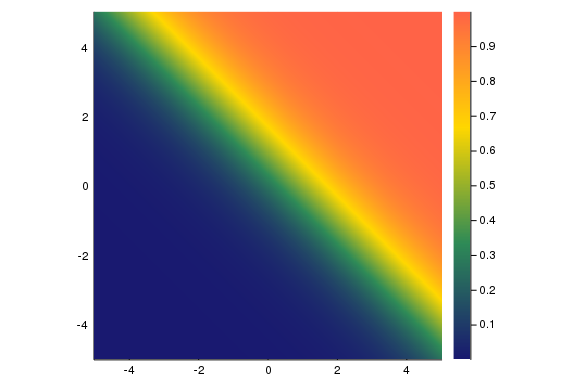samples = [rand(Bool) ? (rand(A),0) : (rand(B),1) for i=1:1000]
cs =  [c for ((x,y),c) in samples]
scatter([(x,y) for ((x,y),c) in samples],group=cs)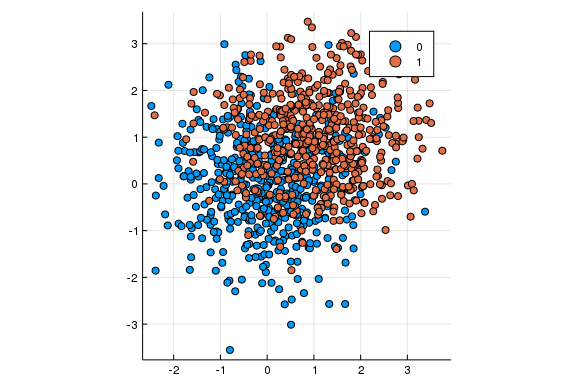σ(u) = 1/(1 + exp(-u))
r(β,x) = σ(β[1;x])
C(β,xᵢ,yᵢ) = yᵢ*log(1/r(β,xᵢ))+(1-yᵢ)*log(1/(1-r(β,xᵢ)))
L(β) = sum(C(β,xᵢ,yᵢ) for (xᵢ,yᵢ) in samples)
β̂ = optimize(L,ones(3),BFGS()).minimizer
r̂s = [r(β̂,[x,y]) for x=xs,y=ys]
using Plots, JuMP, Ipopt, Random; Random.seed!(1234);
gr(aspect_ratio=1)
Plots.GRBackend()
function samplepoint(μ=[3,3])
class = rand([-1,1])
if class == -1
X = randn(2)
else
X = μ + [1 -1/2; -1/2 1] * randn(2)
end
(X,class)
end
n = 100
samples = [samplepoint() for i=1:n]
ys = [y for (x,y) in samples]
scatter([(x₁,x₂) for ((x₁,x₂),y) in samples],group=ys)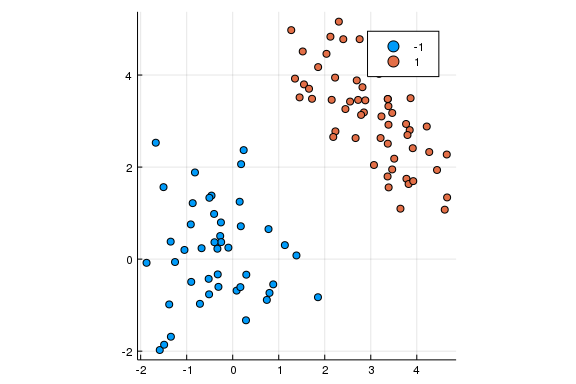m = Model(solver=IpoptSolver(print_level=0))
@variable(m,β[1:2]) # adds β and β at the same time
@variable(m,α)
for (x,y) in samples
@constraint(m,y*(βx - α)  1)
end
@objective(m,Min,β^2+β^2)
β² + β²
solve(m)
β,α = getvalue(β), getvalue(α)
l(x₁) = (α - β*x₁)/β
xs = [-2,5]
plot!(xs,[l(x₁) for x₁ in xs],label="")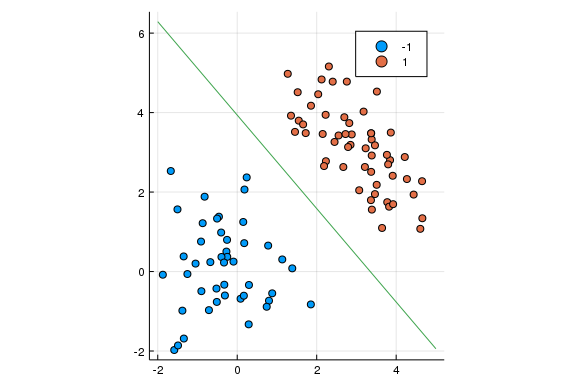samples = [samplepoint([1,1]) for i=1:n]
ys = [y for (x,y) in samples]
scatter([(x₁,x₂) for ((x₁,x₂),y) in samples],group=ys)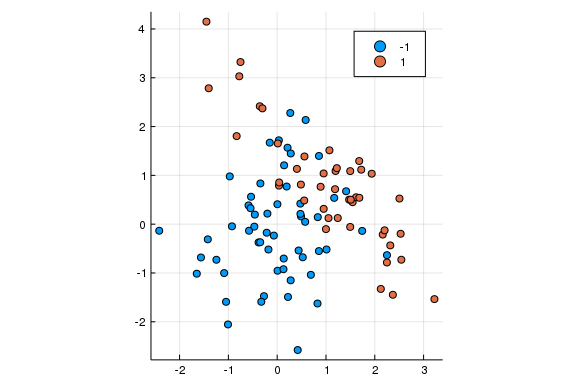L(λ,β,α,samples) = λ*norm(β)^2 + 1/n*sum(max(0,1-y*(βx - α)) for (x,y) in samples)
L(λ,params,samples) = L(λ,params[1:end-1],params[end],samples)
using Optim
function SVM(λ,samples)
params = optimize(params->L(λ,params,samples),ones(3),BFGS()).minimizer
params[1:end-1], params[end]
end
SVM (generic function with 1 method)
function errorrate(β,α,samples)
count(y*(βx-α) < 0 for (x,y) in samples)
end
function CV(λ,samples,i)
β,α = SVM(λ,[samples[1:i-1];samples[i+1:end]])
x,y = samples[i]
y*(βx - α) < 0
end
function CV(λ,samples)
mean(CV(λ,samples,i) for i=1:length(samples))
end
CV (generic function with 2 methods)
λ₁, λ₂ = 0.001, 0.25
λ = optimize(λ->CV(first(λ),samples),
λ₁,λ₂).minimizer
β,α = SVM(λ,samples)
l(x₁) = (α - βx₁)/β
xs = [-1.5,2]
plot!(xs,[l(x₁) for x₁ in xs],label="")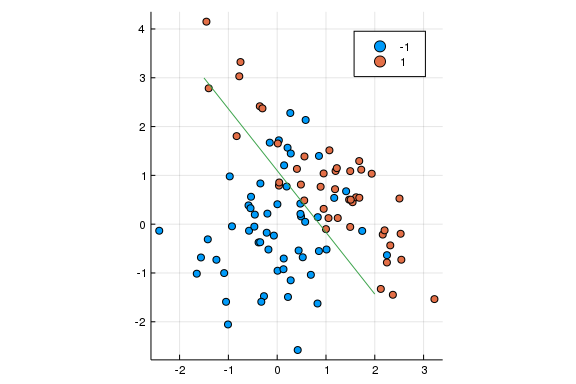struct AffineMap
W::Matrix
b::Vector
end
struct NeuralNet
maps::Vector{AffineMap}
K::Function # activation function
::Function # derivative of K
end
(A::AffineMap)(x) = A.W * x + A.b
(N::NeuralNet)(x) = forwardprop(N,x)[end]
architecture(N::NeuralNet) = [[size(A.W,2) for A in N.maps]; size(last(N.maps).W,1)]
function forwardprop(N::NeuralNet,x)
vectors = [x]
for (j,A) in enumerate(N.maps)
push!(vectors,A(vectors[end]))
push!(vectors,(j < length(N.maps) ? N.K : identity).(vectors[end]))
end
vectors
end
forwardprop (generic function with 1 method)
greennode(j) = 2j-1
purplenode(j) = 2j
function backprop(N::NeuralNet,vectors,yᵢ)
for j = length(N.maps) : -1 : 1
end
end
backprop (generic function with 1 method)
end

end
bias_gradients (generic function with 1 method)
function averageweightΔ(N::NeuralNet,samples,batch,ϵ)
arch = architecture(N)
layers = length(arch)-1
sum_Δweight = [zeros(arch[i+1],arch[i]) for i=1:layers]
sum_Δbias = [zeros(arch[i+1]) for i=1:layers]
for k in batch
x, y = samples[k]
vectors = forwardprop(N,x)
for i=1:layers
sum_Δweight[i] -= ϵ*ΔWs[i]
sum_Δbias[i] -= ϵ*Δbs[i]
end
end
(sum_Δweight, sum_Δbias) ./ length(batch)
end
averageweightΔ (generic function with 1 method)
function train(arch,K,,samples,batchsize,ϵ = 0.1,iterations=1000)
random_maps = [AffineMap(randn(arch[i+1],arch[i]),
fill(0.1,arch[i+1])) for i=1:length(arch)-1]
N = NeuralNet(random_maps,K,)
for i=1:iterations
batch = sample(1:length(samples),batchsize)
meanΔweight, meanΔbias = averageweightΔ(N,samples,batch,ϵ)
N = NeuralNet([AffineMap(A.W .+ ΔW, A.b .+ Δb)
for (A,ΔW,Δb) in zip(N.maps,meanΔweight,meanΔbias)],K,)
end
N
end
train (generic function with 3 methods)
K(x) = x > 0 ? x : 0
(x) = x > 0 ? 1 : 0
cost(N::NeuralNet,samples) = mean(norm(N(x)-y)^2 for (x,y) in samples)
using Random; Random.seed!(123)
xs = [rand(2) for i=1:1000]
ys = [[1-norm(x)^2] for x in xs]
samples = collect(zip(xs,ys));
arch = [2,5,1]
batchsize = 100
ϵ = 0.005
N = train(arch,K,,samples,batchsize,ϵ,10_000)
cost(N,samples) # returns 0.00343
xgrid = 0:1/2^8:1
ygrid = 0:1/2^8:1
zs = [first(N([x,y])) for x=xgrid,y=ygrid]
plotly(); surface(xgrid,ygrid,zs)